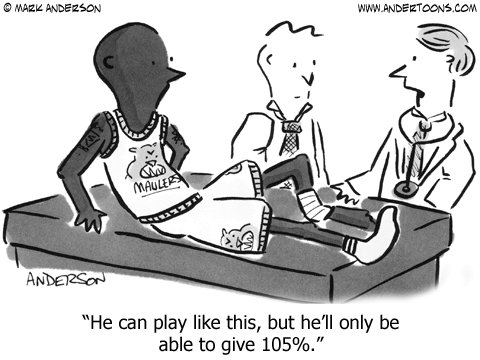## PSA-170830-Mathematical LogicMathematics:

This comes from 2 math teachers with a combined total of 70 yrs. experience.
It has an indisputable mathematical logic.

This is a strictly …..  a mathematical viewpoint… and it goes like this:

What Makes 100%?
What does it mean to give MORE than 100%?
Ever wonder about those people who say they are giving more than 100%? We have all been to those meetings where someone wants you to give over 100%.
What makes up 100% in life?
If:
A B C D E F G H I J K L M N O P Q R S T U V W X Y Z
Is represented as:
1 2 3 4 5 6 7 8 9 10 11 12 13 14 15 16 17 18 19 20 21 22 23 24 25 26.
Then:
H-A-R-D-W-O-R-K
8+1+18+4+23+15+18+11 =  98%
And
K-N-O-W-L-E-D-G-E
11+14+15+23+12+5+4+7+5 =
96%
But ,
A-T-T-I-T-U-D-E
1+20+20+9+20+21+4+5 =  100%
And,
B-U-L-L-S-H-I-T
2+21+12+12+19+8+9+20 =  103%
AND, look how far ass kissing will take you.
A-S-S-K-I-S-S-I-N-G
1+19+19+11+9+19+19+9+14+7  = 118%
So, one can conclude with mathematical certainty, that while Hardwork and Knowledge will get you close, and Attitude will get you there.  It’s the Bullshit and good old fashion Ass Kissing that will put you over the top.
Now you know why Politicians are where they are!
I’ve never seen a better explanation than this formula. How true it is?